LR  >  Bar Chart - Practice Test (With Solutions) CAT, Common Admission Test

# Bar Chart - Practice Test (With Solutions) CAT, Common Admission Test

Test Description

## 13 Questions MCQ Test | Bar Chart - Practice Test (With Solutions) CAT, Common Admission Test

Bar Chart - Practice Test (With Solutions) CAT, Common Admission Test for LR 2023 is part of LR preparation. The Bar Chart - Practice Test (With Solutions) CAT, Common Admission Test questions and answers have been prepared according to the LR exam syllabus.The Bar Chart - Practice Test (With Solutions) CAT, Common Admission Test MCQs are made for LR 2023 Exam. Find important definitions, questions, notes, meanings, examples, exercises, MCQs and online tests for Bar Chart - Practice Test (With Solutions) CAT, Common Admission Test below.
Solutions of Bar Chart - Practice Test (With Solutions) CAT, Common Admission Test questions in English are available as part of our course for LR & Bar Chart - Practice Test (With Solutions) CAT, Common Admission Test solutions in Hindi for LR course. Download more important topics, notes, lectures and mock test series for LR Exam by signing up for free. Attempt Bar Chart - Practice Test (With Solutions) CAT, Common Admission Test | 13 questions in 26 minutes | Mock test for LR preparation | Free important questions MCQ to study for LR Exam | Download free PDF with solutions
 1 Crore+ students have signed up on EduRev. Have you?
Bar Chart - Practice Test (With Solutions) CAT, Common Admission Test - Question 1

### The following bar chart shows the monthly expenditure of a family comprising of five persons over a period of seven months during three different years.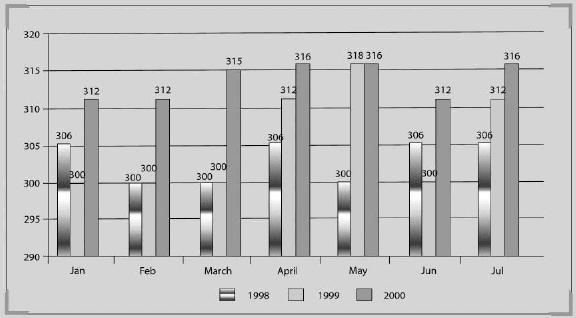What is the average monthly expenditure of the whole family in the year 2000?

Bar Chart - Practice Test (With Solutions) CAT, Common Admission Test - Question 2

### The following bar chart shows the monthly expenditure of a family comprising of five persons over a period of seven months during three different years.Which period has shown the same change in trend across all the given three years?

Detailed Solution for Bar Chart - Practice Test (With Solutions) CAT, Common Admission Test - Question 2

The same increasing trend is seen every year during the period March-April.

Bar Chart - Practice Test (With Solutions) CAT, Common Admission Test - Question 3

### For how many months of the given years the expenditure has been consistently increasing or decreasing?

Detailed Solution for Bar Chart - Practice Test (With Solutions) CAT, Common Admission Test - Question 3

Only April satisfies these conditions.

Bar Chart - Practice Test (With Solutions) CAT, Common Admission Test - Question 4

Refer to the following bar graph and solve the based on it.
The bar chart given below represents top 10 exporters and their export value (in billion \$) in 2008.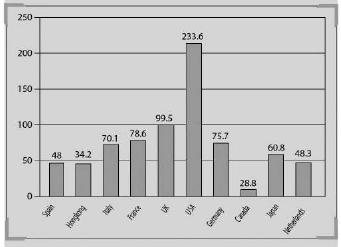If India also joins the top ten exporters list by becoming the 11th largest exporter, then what will be the effect on the average exports of these 11 countries; provided the export value of India is \$ 10 billion?

Detailed Solution for Bar Chart - Practice Test (With Solutions) CAT, Common Admission Test - Question 4

The average will decrease by 6.11 billion dollars. Hence, option (d) is the correct answer.

Bar Chart - Practice Test (With Solutions) CAT, Common Admission Test - Question 5

Refer to the following bar graph and solve the based on it.
The bar chart given below represents top 10 exporters and their export value (in billion \$) in 2008.What is the average export of all the countries in billion dollars for the year 2008?

Detailed Solution for Bar Chart - Practice Test (With Solutions) CAT, Common Admission Test - Question 5

Cannot be determined because the data is given only for the top ten exporters.

Bar Chart - Practice Test (With Solutions) CAT, Common Admission Test - Question 6

Refer to the following bar graph and solve the based on it.
The bar chart given below represents top 10 exporters and their export value (in billion \$) in 2008.If the average export of the top ten exporters is calculated how many of the top ten exporting countries have higher export than this average?

Detailed Solution for Bar Chart - Practice Test (With Solutions) CAT, Common Admission Test - Question 6

The average is 77.6. Now it can be seen that only three countries have more than this.

Bar Chart - Practice Test (With Solutions) CAT, Common Admission Test - Question 7

Refer to the following bar graph end solve the questions based on it.
Amount of production and sales by a company over the years (in lacs tonnes)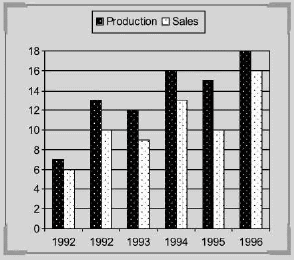What is the difference between the sales of 1993 and 1995 (in thousand tonnes)?

Detailed Solution for Bar Chart - Practice Test (With Solutions) CAT, Common Admission Test - Question 7

The difference between sales of 1993 and 1995 = 1 lacs or 100,000

Bar Chart - Practice Test (With Solutions) CAT, Common Admission Test - Question 8

Refer to the following bar graph end solve the questions based on it.
Amount of production and sales by a company over the years (in lacs tonnes)What is the approximate percentage increase in production from 1993 to 1994?

Detailed Solution for Bar Chart - Practice Test (With Solutions) CAT, Common Admission Test - Question 8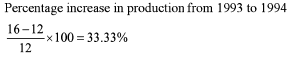Bar Chart - Practice Test (With Solutions) CAT, Common Admission Test - Question 9

Refer to the following bar graph end solve the questions based on it.
Amount of production and sales by a company over the years (in lacs tonnes)What is the percentage drop in sales from 1992 to 1993?

Detailed Solution for Bar Chart - Practice Test (With Solutions) CAT, Common Admission Test - Question 9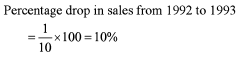Bar Chart - Practice Test (With Solutions) CAT, Common Admission Test - Question 10

Refer to the following bar graph and solve the questions based on it.
The following bar chart gives the cumulative percentage of five different types of bikes produced by Kajaj during tiie given two years: Total Bikes produced in 2006 is 4,50,000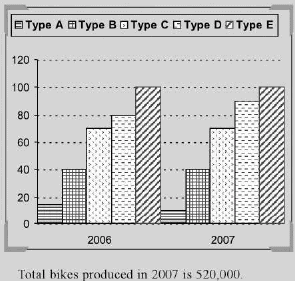If 85% of the E type bikes produced during 2006 and 2007 are being sold by the company, then how many E type bikes are left unsold?

Detailed Solution for Bar Chart - Practice Test (With Solutions) CAT, Common Admission Test - Question 10

Production of E type bikes in 2006

= (100-80)% of 4,50,000 = 20% of 4,50,000 = 90,000

And in 2007 = 10% of 5,20,000 = 52,000

Total production = 90,000 + 52,000  = 1,42,000

So, the number of bikes

= 15% of 1,42,000 = 21,300

Bar Chart - Practice Test (With Solutions) CAT, Common Admission Test - Question 11

Refer to the following bar graph and solve the questions based on it.
The following bar chart gives the cumulative percentage of five different types of bikes produced by Kajaj during tiie given two years: Total Bikes produced in 2006 is 4,50,000Production of B type bikes in 2007 is what percentage of bikes produced in 2006?

Detailed Solution for Bar Chart - Practice Test (With Solutions) CAT, Common Admission Test - Question 11

Percentage production of B type bikes in 2007 = that in 2006 (given) = (40 - 15) = 25% of 5,20,000 = 1,30,000.

Bar Chart - Practice Test (With Solutions) CAT, Common Admission Test - Question 12

Refer to the following bar graph and solve the based on it.
The bar graph given below shows the five districts of the state of Himachal Pradesh and the number of tourists visiting them. For the given bar chart, total number of Indian tourists is more that the total number of foreign tourists. None of the tourists can be Indian as w^ell as foreigner.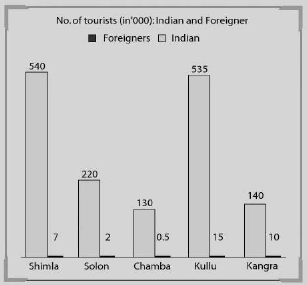Approximately what percentage of the total tourists visiting Himachal Pradesh are foreigners?

Detailed Solution for Bar Chart - Practice Test (With Solutions) CAT, Common Admission Test - Question 12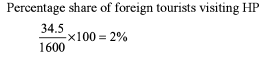Bar Chart - Practice Test (With Solutions) CAT, Common Admission Test - Question 13

Refer to the following bar graph and solve the questions based on it, The following bar charts represents the value of exports and imports (in Rs crores) of n country for the given period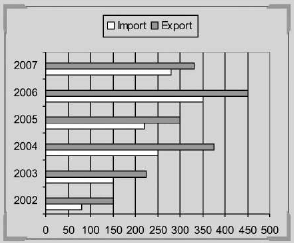(All the values in the above bar chart are the multiples of 10).

The value of exports in 2006 was what percentage of the average value of imports in the years 2004, 2005 and 2007?

Detailed Solution for Bar Chart - Practice Test (With Solutions) CAT, Common Admission Test - Question 13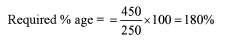Use Code STAYHOME200 and get INR 200 additional OFF Use Coupon Code
Information about Bar Chart - Practice Test (With Solutions) CAT, Common Admission Test Page
In this test you can find the Exam questions for Bar Chart - Practice Test (With Solutions) CAT, Common Admission Test solved & explained in the simplest way possible. Besides giving Questions and answers for Bar Chart - Practice Test (With Solutions) CAT, Common Admission Test, EduRev gives you an ample number of Online tests for practice